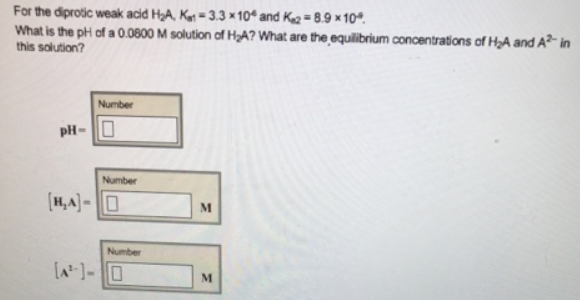# Problem: For the diprotic weak acid H2A, Ka1 = 33 x 10-4 and Ka2 = 89 x10-4.What is the pH of a 0.0800 M solution of H 2A? What are the equilibrium concentrations of H 2A and A2- in solution?

###### FREE Expert Solution
99% (424 ratings)###### Problem Details

For the diprotic weak acid H2A, Ka1 = 33 x 10-4 and Ka2 = 89 x10-4.

What is the pH of a 0.0800 M solution of H 2A?

What are the equilibrium concentrations of H 2A and A2- in solution?